David A. Kenny
September 9, 2011
Page being revised.

Psychometrics

Standard Formulation
Model: Score = True Score plus Error or X = T + E

Note that T and E have implicit coefficients of 1

Assumptions
Average error of zero
True score and error uncorrelated

Example
Mothers report of childs aggression (X) is determined by true aggression (T) and error (E).  Notice that the paths are both 1 and the E and T are uncorrelated: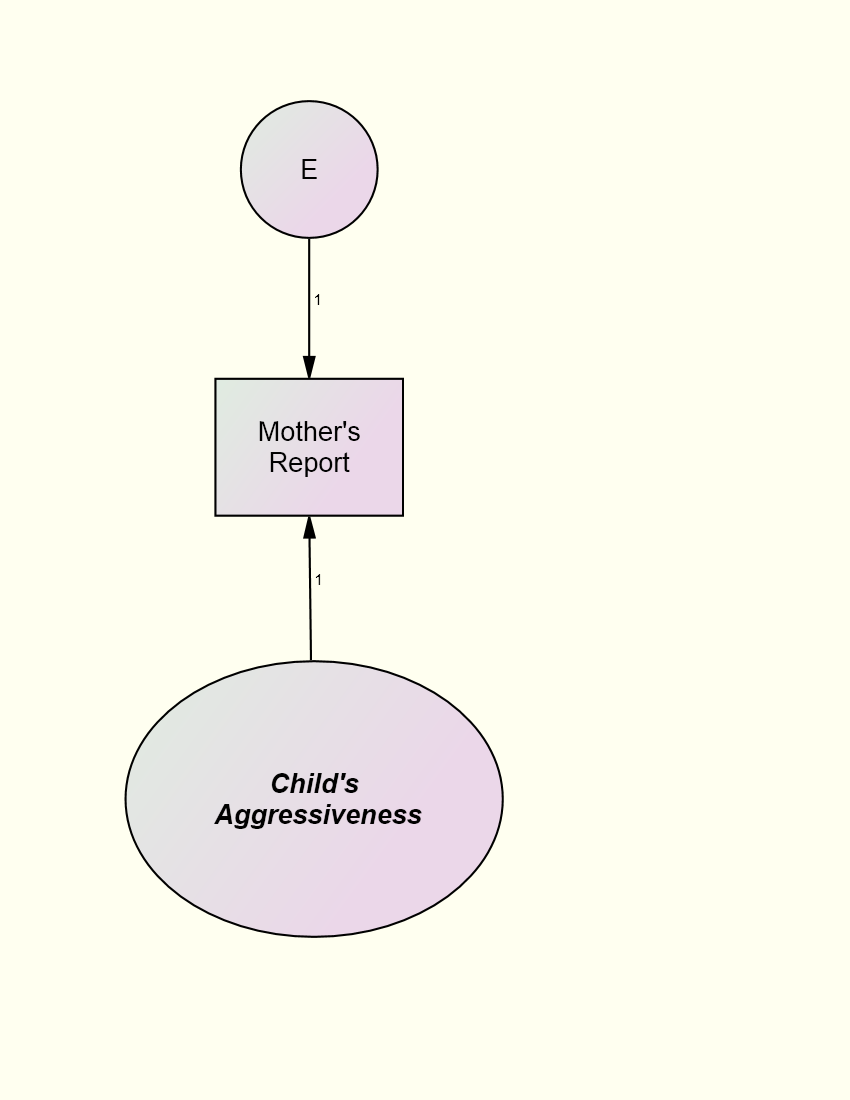Definition of Components
Classical
True score: the meaningful portion of variance or the average of all possible measures of the true score
Measurement error: irrelevant sources of variance or the score minus the true score.
Modern (i.e., Generalizability Theory)
The variance of the score consists of many different components.  In a particular research context, some of these components are meaningful (the true score in that context) and others are not (error in that context).

Reliability

The proportion of variance due to true score or V(T)/V(X) where "V" means variance.

Do not confuse reliability with how to measure it (e.g., internal consistency or test-retest).  Reliability refers not just to the measure, but to sample and context of measurement.  Thus, with a different sample or context, it can change.

Use RELCOMP, a computer program for reliability computation.

Standardized Model

If all the variables are standarized the X = aT +bE.
The path from the true score or T to measured variable or X when both variables are standardized equals the square root of the reliablity. Thus, the correlation of the measure with the true score equals the square root of the reliablity.

Reliability Estimation
Is the score repeatable?
Different Measures (or Observers): Internal Consistency
Standard measure: Cronbach's alpha
Formula: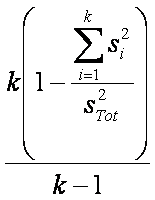Different Times
Test-retest correlation (Presumes no change in the true score)

Classical Assumptions
Equations
X1 = T + E1
X2 = T + E2

Parallel Measures
Equal True Score Variance (both weights for T are 1)
Equal Error Variance:  V(E1) = V(E2)
Error Variance Is Independent across Measures rE1,E2 = 0

Given Classical Assumptions: Reliability = Correlation between Measures

Example: To measure the reliability of mothers or fathers report correlate the two variables.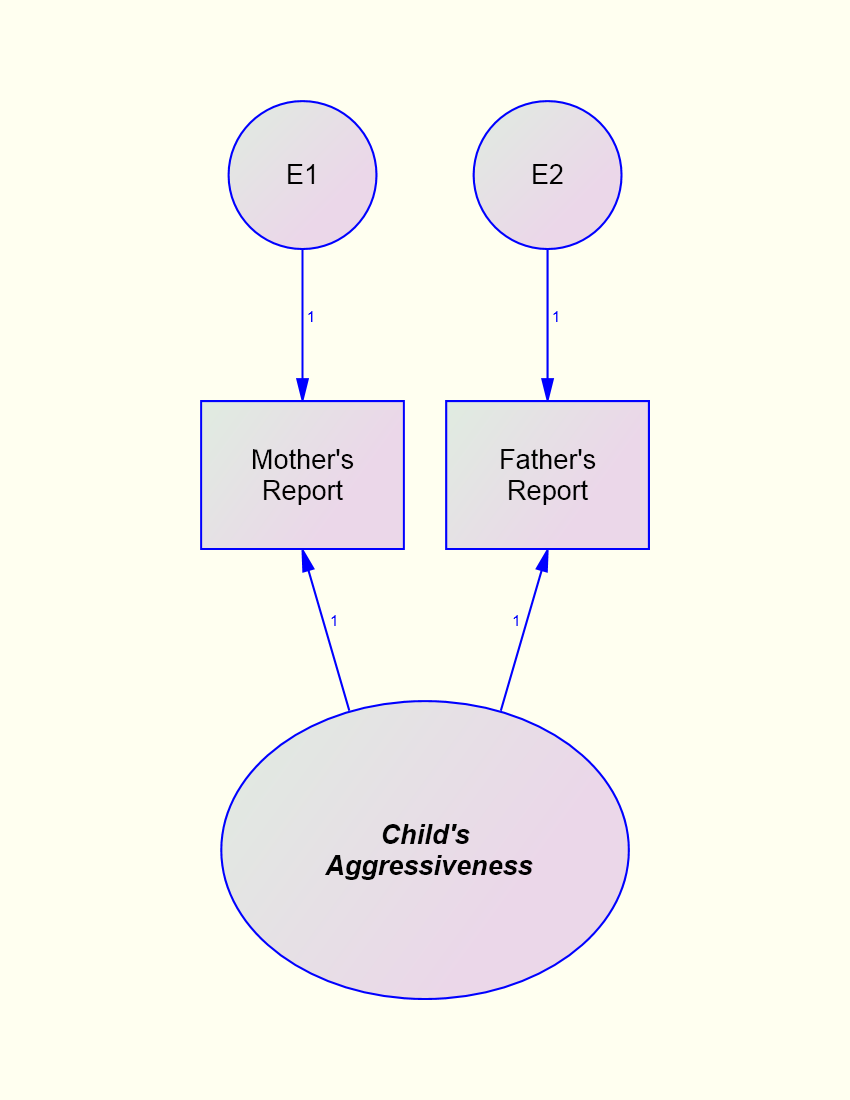Spearman-Brown Profecy Formula
If the average inter-item correlation or r is known, it can be used to forecast the reliability of a test with k items:This formula gives Cronbach's alpha if all the measures had equal variance which would happen if all the measures were standardized.  This formula has many uses.  It can be used to determine the reliability of a test if more or less items are used.  For instance, if a test has 12 items and an average inter-item correlation of .2, then its reliability is .75.  But if the test were to have 24 items, its reliability would be .86 and with 6 items the reliability would be .60.

Another useful formula, again derived from the Spearman-Brown, is estimate of the average inter-item correlation if we know alpha or a and the number of items or k.  The formula is

``

The average inter-item correlation tends to be smaller than you might think.  For instance, the average inter-item correlation for the Peabody Picture Vocabulary Test is about .08, the Rosenberg Self-Esteem Inventory is about .34, and the Beck Depression Inventory is about .26.

Correction for Attenuation

To determine what the correlation between the two variables would be if the variables' reliabilities were perfect, a correlation needs to be divided by the square root of the product of the two variables' reliability.  It is in essence, a forecast of what the correlation would be if the two variables were measured with many (i.e., infinite) items.  The formula is

``

where rXX and rYY are the reliabilities of X and Y, respectively.  So if the reliabilities of X and Y are both .8, and the correlation between X and Y is .4, then the corrected for attenuation correlation is .5.  A disattenuated correlation is not an ordinary Pearson correlation and its range is not +1 to -1.One cannot perform the usual significance test on these correlations.  Also one should avoid disattenuating a correlation if the reliabilities are small to avoid wild values.

SEM Terminology
Indicator: a measure in structural model than contains measurement error; usually represented by a box

Construct or latent variable: a theoretical variable in a model which is tapped by indicators; usually represented by an oval

Relaxing the Assumptions of Classical Test Theory
Correlated errors:  Classical theory sets all correlations between error to zero.  However, two indicators of the same construct may share variance because they are measured by a common method.  That correlation is represented by a curved line in a path diagram.

Unequal erro variances:  Classical theory sets the error variances to b equal, but this assumption can be relaxed.

Estimated True Score
The estimate of th true score given X is
`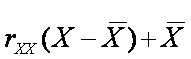`

where rXX is the reliability of X.  The estimate is said to be shrunken or regressed to its mean.

The Effects of Unreliability in Causal Models

If a causal variable has measurement error, the estimate of its effect is biased, as well as the effects of other variables in the structural equation.  Measurement error in the effect variable does not bias its coefficient unless the variables are standardized.  In this case the bias is that the true beta equal the measured beta divided by the square root of the endogenous variable's reliability.  For a causal variable X, measurement error biases the estimate of another causal variable Z that is in the equation when:
Causal variable X has measurement error.
Variables X and Z are correlated.
X affects the endogenous variable.

These three factors multiply to produce bias and so if any one is missing, there is no bias.

Correcting for the Effects due to Measurement Error
There are three ways to remove the biasing effects of unreliability i the causal variable:
Instrumental Variable Estimation
Multiple Indicators (Latent Variable Models)
The last strategy is problematic because it presumes that reliabilities are exactly known which is never true and sometimes estimation breaks down because the correlation matrix is ill-conditioned.  To implement the strategy, one would input the correlation or covariance matrix and fix the measure's error variance to one minus reliability for the correlation matrix and (1 - reliability) times the measure's variance for the covariance matrix.  Later on the SEM page, another and better correction for attenuation strategy is presented (go to that page).

Item Response Theory
What has been presented above is sometimes called classical test theory (CTT).  A modern alternative to CTT is item response theory which presumes a dichotomous response.  Item response theory is very complicated and highly mathematical.

Go to the next SEM page.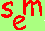Go to the main SEM page.